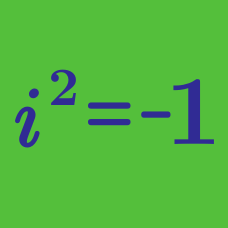Algebra

# Complex Numbers Problem Solving

Let $z = a^2 - 5a - 36 + (a^2 + 6a + 8)i$. What is the sum of all real numbers $a$ such that $z^2$ is a negative real number?

Details and assumptions

$i$ is the imaginary number that satisfies $i^2 = -1$.

If the quadratic equation $(2 + i)x^2 + (a - i)x + 46 - 2i = 0$ has real roots, what is the value of the positive real constant $a$?

Details and assumptions

$i$ is the imaginary number that satisfies $i^2 = -1$.

Let $z=x(1-i)+8(-2+i),$ where $x$ is a real number. If $z^2$ is a negative real number, what is the value of $x?$

Real numbers $x$ and $y$ satisfy the equation: $\frac{x}{1+i} + \frac{y}{1-i} = \frac{148}{12 + 2i}.$ What is the value of $xy?$

Details and assumptions

$i$ is the imaginary number satisfying $i^2 = -1$.

$\frac{12 - \sqrt{-9}}{12 + \sqrt{-9}} + \frac{12 + \sqrt{-9}}{12 - \sqrt{-9}}$ can be simplified to $\frac{a}{b}$, where $a$ and $b$ are coprime positive integers. What is the value of $a + b$?

×